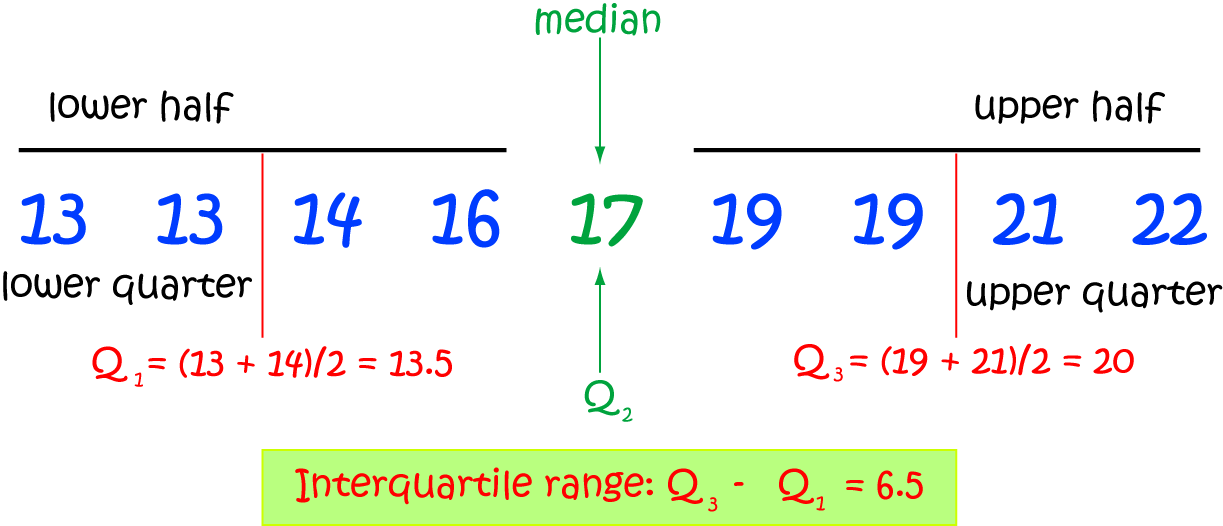Pull Down & Release to Refresh

# Definition of QuartilesQuartiles are numbers that divide a set of data into four equal parts.

To find the quartiles, you need to first write the numbers in your data set in increasing order.

You then find the middle value or median. This is equal to the second quartile, $Q_2$, and divides the set of numbers into two halves.

Next you find the middle values of each of the two halves. The middle value of the lower half is the first quartile, $Q_1$. The middle value of the upper half is the third quartile, $Q_3$.

If any part of the list has two middle values, the quartile is found by adding them together and dividing the sum by $2$.

### Description

The aim of this dictionary is to provide definitions to common mathematical terms. Students learn a new math skill every week at school, sometimes just before they start a new skill, if they want to look at what a specific term means, this is where this dictionary will become handy and a go-to guide for a student.

### Audience

Year 1 to Year 12 students

### Learning Objectives

Learn common math terms starting with letter Q

Author: Subject Coach
You must be logged in as Student to ask a Question.